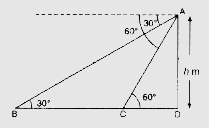# A straight highway leads to the foot of a tower. A man standing at the top of the tower observes a car at an angle of depression of $30^o$, which is approaching the foot of the tower with a uniform speed. Six seconds later, the angle of depression of the car is found to be $60^o$. Find the time taken by the car to reach the foot of the tower from this point.

Given:

A straight highway leads to the foot of a tower.

A man standing at the top of the tower observes a car at an angle of depression of $30^o$, which is approaching the foot of the tower with a uniform speed.

Six seconds later, the angle of depression of the car is found to be $60^o$.

To do:

We have to find the time taken by the car to reach the foot of the tower from this point.

Solution:Let $BCD$ be the highway.

The tower of height $h$ is standing at point $D$.

From the top of the tower of point $A$ the angle of depression is $30^{\circ}$.

After 6 sec when car reaches the point $C$ then angle of depression becomes $60^{\circ}$.

This implies,

The distance covered in $6 \mathrm{sec}=\mathrm{BC}$

From right angled triangle $\mathrm{ADB}$,

$\tan 30^{\circ}=\frac{\mathrm{AD}}{\mathrm{BD}}$

$\frac{1}{\sqrt{3}}=\frac{h}{BD}$

$BD=h \sqrt{3}$.............(i)

From right angled triangle $ADC$,

$\tan 60^{\circ}=\frac{\mathrm{AD}}{\mathrm{CD}}$

$\sqrt{3}=\frac{h}{CD}$

$h=\sqrt{3}CD$.........(ii)

Substituting the value of $h$ in (i), we get,

$BD=\sqrt{3}CD \times \sqrt{3}$

$=3 C D$

$BC+CD=3CD$

$3CD-CD=BC$

$2CD=BC$

$CD=\frac{1}{2} \mathrm{BC}$

The time taken to cover the distance $CD=\frac{1}{2} \times$ Time taken to cover distance $BC$

$=\frac{1}{2} \times 6$

$=3$

Therefore, the time taken by the car to reach the foot of the tower from this point is 3 seconds.